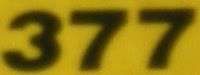## Friday, December 17, 2010

### 377

377 = 13 x 29.

377 has two representations as a sum of two squares: 377 = 42 + 192 = 112 + 162.

377 is the fourteenth Fibonacci number. It is the largest known Fibonacci number whose digits are all prime.

377 is the hypotenuse of two primitive Pythagorean triples: 3772 = 1352 + 3522 = 1522 + 3452.

377 is the sum of the squares of the first six primes: 377 = 22 + 32 + 52 + 72 + 112 + 132.The Aero Spacelines B-377 SG Super Guppy is a four-engine oversize-cargo freighter aircraft.